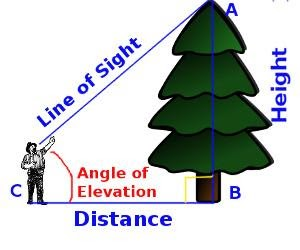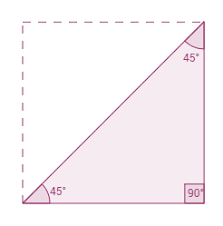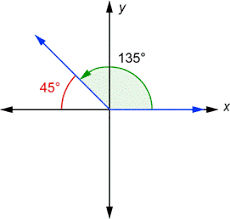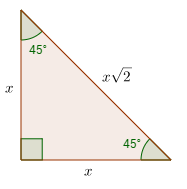Learn US

# Sin pi/4, cos pi/4, tan pi/4, cot pi/4, sec pi/4, cosec pi/4

1k views

 1 Introduction 2 Sin 45 3 Cos 45Few tips every student should follow to improve results 4 Tan 45 5 Cot 45 6 Cosec 45 7 Sec 45 8 Summary

21th September 2020

## IntroductionIt is really difficult to learn the ratios as a student when you are first introdu

ced to trigonometry, but by just understanding the concept behind it I am sure you won’t forget the ratios I am mentioning here. I will be explaining the values of Sin 45, cos 45, and Tan 45, etc.

Trigonometry as the name suggests deals about triangles and that too right triangles are the most important. Why?

If we know the hypotenuse and the distance and the angle of elevation we can find the height of the tree using Trigonometry. Questions like this are common in many fields and trigonometry gives us the answers.

The main functions of trigonometry are sine cosine and tangent.

For any angle theta just remember the mnemonic  SOHCAHTOA

”SOH” – Sine is Opposite over Hypotenuse, "CAH" - meaning Cosine is Adjacent over Hypotenuse. ”TOA” – Tangent is Opposite over Adjacent.

and their reciprocals can also be deduced in the same manner for cosec x, sec x and cot x

No based on the formulas above how to find out sin 45, cos 45, and Tan 45.Consider the right triangle ABC which is right-angled at B, angle C is 45 ̊. Since the triangle is Isosceles the sides AB and AC are equal. Let us go with the unit triangle and suppose that AB and BC are of measure 1 unit. Now,

applying the Pythagorean theorem we can calculate the hypotenuse AC

AC² = AB² + BC²

AC² = 1² + 1²

AC² = 2

## Sin 45 or sin pi/4

The trigonometric function is also known as a trigonometric ratio or an angle function is used to relate the angles of a triangle to its sides.

### Sin 45 Value

The value of the sin 45 degrees or sin pi/4 is 1/√2

Sin 45° = 1/√2 is an irrational number and it equals 0.7071067812 approx.

For angles less than a right angle, trigonometric functions are commonly defined as the ratio of two sides of a right triangle. Here, we will discuss the value for sin 45 degrees and we were taught how to derive the sin 45 value using other degrees or radians.

### Geometrically Derivation of sine 45In ΔABC,

AC=√2

we get for the values of 45 degrees, the opposite is 1 unit, the hypotenuse is AC which is √2 units and adjacent is 1 unit by Pythagoras theorem.

Therefore sin 45 =1/√2=√2/2

Sin 45=√2/2

which is equal to 0.7071067812

### Why is sin 45 equal to sin 135?

The value of sin 45 degrees = sin 135 degrees

Sin 45 = sin 135 = 1/√2Both are equal because the reference angle for 135 is equal to 45 for the triangle formed in the unit circle. The reference angle is formed when the perpendicular is dropped from the unit circle to the x-axis, which forms a right triangle.

Since the angle 135 degrees lies in the IInd quadrant, therefore the value of sin 135 is positive. The internal angle of the triangle is 180 – 135 = 45, which is the reference angle.

### Sin(-45)

sin(-x) = -sin(x)  (odd function)

Hence, sin (-45) = -sin 45

sin(-45) = -1/√2

## Cos 45 or cos pi/4

In trigonometry, the cosine function is defined as the ratio of the adjacent side to the hypotenuse

### Cos 45 value

The value of Cos 45 degree is 1/√2

Another Different form of Cos 45° is cos pi/4 or cos π/4

Hence, cos pi/4 =  1/√2

### Geometrically Derivation of cosine 45

Now we calculate the value of Cos 45 degrees through a practical approach. So if we drew the right triangle APS using constructions,

Cos pi/4 can be calculated as √½

OR

Using Pythagorean identity, we get

sin245°+ cos245°=1

cos245°=1 -sin245°

cos245°=1- 1√22

cos245°=  1- 12=  12

### Cos 135?

Since cos (180-x) = -cos x,

cos 135 = - cos 45

cos 135 = cos 3pi/4 = -1/√2

### Cos(-45)

cos(-x) = cos(x)  (even function)

Hence, cos (-45) = cos 45

cos(-45) = 1/√2

## Tan 45 or tan pi/4

In trigonometry, the tangent of an angle in a right-angled triangle is equivalent to the ratio of the opposite side and the adjacent side.

### Tan 45 value

The value of tan 45 degrees is 1

Tan 45 degrees is also expressed by tan π/4 in terms of radians.

Hence, tan pi/4 is also 1

The value of the tangent of angle 45 degrees can also be evaluated using the ratio of the values of sin 45 degrees and cos 45 degrees.

### Geometrically Derivation of Tangent 45

Now we can find tan 45 Value Using the Sine function and Cos Function

We can also represent the tangent function as the ratio of the sine function and cosine function.

∴ Tan x = sin x/cos x

So, tan 45 degrees we can expressed as ;

Tan 45° = sin 45°/cos 45°

We know,

Sin 45° = 1/√2 &  Cos 45° = 1/√2
∴ Tan 45° = (1/√2)/(1/√2)

Tan 45° = 1

So, the value of Tan 45 degrees or tan pi/4 is 1

### Tan(-45)

tan(-x) = -tan(x)  (odd function)

Hence, tan(-45) = -tan45

tan(-45) = -1

## Cot 45 or cot pi/4

In trigonometry, the Cotangent function is defined as the ratio of the adjacent side to the Opposite side.

### Cot 45 value

The value of the Cot 45 degree or cot pi/4 is 1

### Derivation of Cot pi/4

cot  x = 1/tan x.

Using this identity, we can find cot pi/4

cot pi/4 = 1/tan pi/4

Therefore, cot 45 or cot pi/4 is 1

### Cot(-45)

cot(-x) = -cot(x)  (odd function)

Hence, cot(-45) = -cot45

cot(-45) = -1

## Cosec pi/4 or cosec 45

The cosecant ratio of an angle is the length of the hypotenuse divided by the opposite side.

it is abbreviated to just csc.

### Value of Cosec 45 or Cosec pi/4

The value of cosec 45 degrees or cosec pi/4 is √2

It can be derived as the reciprocal of sine:

Cosec = 1/Sine

The approx value of cosec 45° is 1.414

### Geometrically Derivation of Cosecant 45cosec 45 = 1/sin 45

Hence, the value of cosec 45 is √2.

## Secant 45 degree

The secant function defines a relation between the hypotenuse and the adjacent side of the right-angled triangle.

### Value of sec 45 or sec pi/4

The value of Sec 45 degrees is √2.The secant function is the transpose of the cosine function

### Geometrically Derivation of Secant 45

= Hypotenuse/Base
sec x = h/b

Now, sec ∠A = 1/cos ∠A

Therefore, sec 45 = 1/cos 45

sec 45 = 1/cos 45

Therefore,

sec 45 or sec pi/4 is √2

There is yet another way of finding out the values of all trigonometric ratios. For learning the Trigonometric values table much quicker please visit: Trigonometry TableNow using this formula, say we want to know the height of a tree where the Angle of elevation is 45 ̊

And the hypotenuse is 30 units

Sin 45=opposite/hypotenuse

The opposite (height of the tree) is = 30×0.7071.. = 21 units approximately This is the height of the tree.

### Practice Time

To get a deep knowledge of Trigonometry, try out the following problems. You may use the formulas and tables given in this article.

Q.1: In △ABC, right-angled at B, AB =1 cm and BC = 1 cm. Find the angles A and C and hence evaluate:

(a) sin A Cos B            (b) tan A tan B

Q.2: If cot θ= 1, then find sec θ.

## Summary

I hope this article has helped you to understand trigonometric angles of 45 deg or  3 radians. In higher classes, you will learn that angles may vary as any real value. And if we know the value of basic angles then many other values can be calculated in a fraction of seconds.

 𝚹 (°) 45° 𝚹(rad) π/3 Sine 1/√2 Cosine 1 /√2 Tan 1 Cot 1 Cosec √2 Sec √2

GIVE YOUR CHILD THE CUEMATH EDGE
Access Personalised Math learning through interactive worksheets, gamified concepts and grade-wise courses